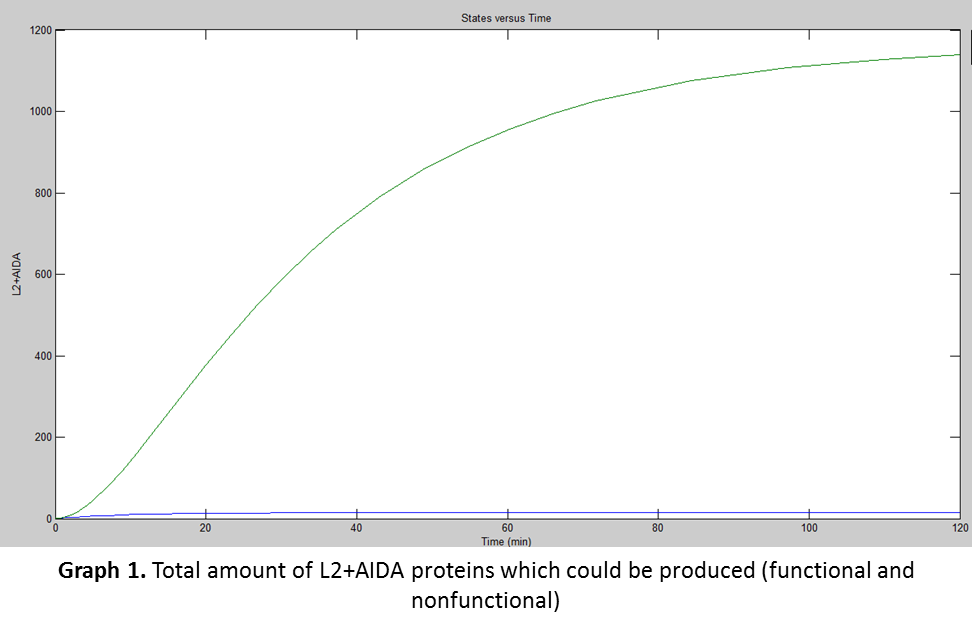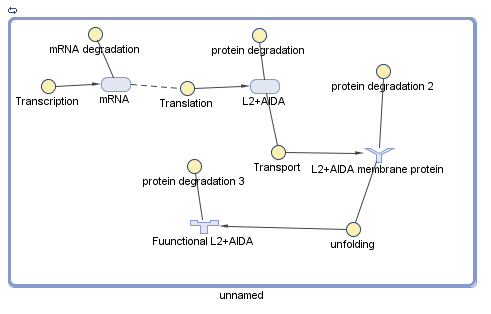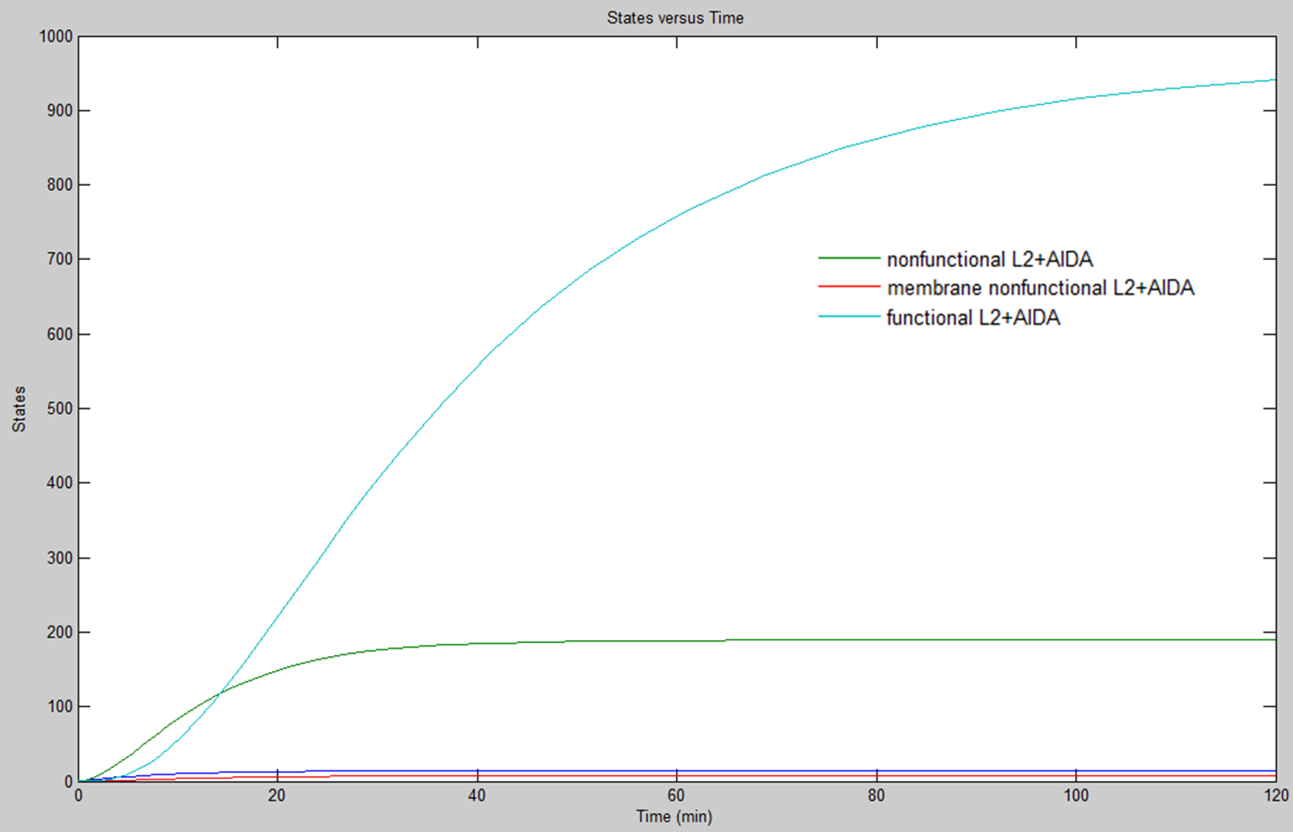# Team:CIDEB-UANL Mexico/math union

iGEM CIDEB 2014 - Project

## Union Module

The union module is based on the use of a fusion protein composed by L2 and AIDA. L2+AIDA is a protein which is not affected by external factors during its transcription or in its translation, so the established parameters were needed to use:

\begin{equation} \large \frac{d\left [ mRNA \right ]}{dt}= \alpha_{1}-d_{1}\left [ mRNA \right ] \end{equation}

The parameters for translation and transcription rates from Singapore 2008 iGEM team were used, as well as the speeds at which E. coli carried out the transcription and translation, assuming a transcription speed of 70nt/s and a translation speed of 40aa/s. These data were used in the equations below with the L2+AIDA gene length (2620nt) and protein length (856aa) respectively:

\begin{equation} \large \alpha_{1} = \frac{transcription speed}{gene length (nt)} \end{equation}
\begin{equation} \large \alpha_{2} = \frac{translation speed}{protein length (aa)} \end{equation}
\begin{equation} \large \alpha_{1} = \frac{(70)(60)}{2620} = 1.6 \end{equation}
\begin{equation} \large \alpha_{2} = \frac{(40)(60)}{856} = 2.8 \end{equation}

After, it was needed to use the parameters for degradation rates of proteins and mRNAs obtained from Beijing PKU 2009 iGEM team:

\begin{equation} \large d_{1} = \frac{1}{half-life(min)} + \frac{1}{30min} \end{equation}
\begin{equation} \large d_{2} = \frac{1}{half-life(min)} + \frac{1}{30min} \end{equation}

Since it was a fusion protein, a research was made to find out the half-life of each protein. The half-life of membrane proteins range between 2 to 20 hours in E. coli (Hare, 1991), and as AIDA-I is a membrane protein its half-life must be between that range, but it was not obtained the specific AIDA’s half-life. L2’s half-life was assumed to be 7.8 hours (Bergant, 2010). Bergant’s team made a test with a homologous protein, but found in the minor capsid of the Human Papillomavirus (HPV). Although the function of the L2 strand in HPV is viral and in E. coli is ribosomal, both share similar structures and sequences. Once it was decided to use the half-life from the homologous L2, it was used as the half-life for the fusion protein because it was between the range of AIDA-I and also because it was the lower half-life, assuming when E. coli starts L2 degradation, the whole protein would be degraded.

Later, was used the information from Selinger’s team (2003) to determine the mRNA degradation. They performed several experiments for finding the average mRNA half-life in E. coli. They used mRNAs about 1100nt concluding they have an average half-life of 5min. Using the previous relation was found mRNA’s half-life from L2+AIDA which was about 11.9min.

\begin{equation} \large HL = \frac{1100(nt)}{5 min} \end{equation}

With all these information, the degradation rates for both transcription and translation of L2+AIDA were found:

\begin{equation} \large d_{1} = \frac{1}{11.9} + \frac{1}{30} = 0.11 \end{equation}
\begin{equation} \large d_{2} = \frac{1}{468} + \frac{1}{30} = 0.035 \end{equation}

Simbiology® was used for the simulation. The previous data from the equations were used to calculate the amount of proteins E. coli would produce at certain time. The results obtained are shown in the following graph:For translation there was another factor that was needed to be taken into consideration, the “fpost, which were the post-translational variables affecting the production of the functional protein:
\begin{equation} \large \frac{d[P]}{dt} = \alpha_{2} \cdot[mRNA] - d_{2}[P] - f_{post} \end{equation}

Since the fusion protein needs to be expressed in E. coli’s membrane, it was needed to find the average velocity at which E. coli exports proteins to its membrane. The process by which bacteria exports its proteins are divided into three phases: the “breathing” (which happens between translation and the second phase), the second phase (which is protein movement), and translocation (in this phase the protein attaches to the membrane of the bacteria) (Peskin, 1991).With the previous data it was determined E. coli completes these phases in an average of 5 to 6 min, depending on the protein size (Driessen, 1990). The time used was 5.5min because L2+AIDA is not too big (2620nt).

According to Ikeda and Kuroda (2011), L2 carries out an unfolding process to become functional . It was found that 50S ribosomal proteins L2, L3, L14, L23, L24, and L32, as well as the 30S ribosomal proteins, S12 and S18 are native premolten globules in their free forms, but adopted rigid well-folded conformations during the formation of a functional ribosome. They exhibit several amounts of ordered secondary structures; the unfolding of a protein molecule results in an essential increase in its hydrodynamic volume. For instance, there is a well-documented 15–20% increase in the hydrodynamic radius of globular proteins upon their transformation into the molten globule state (Unversky, 2002). Also, Unversky developed an equation used to determine unfolding rates shown next:

\begin{equation} \large [H]boundary = \frac{[R]+1.51}{2.785} \end{equation}

This equation gives the estimation of the "boundary" mean hydrophobicity value, “[H]boundary”, below which a polypeptide chain with a given net charge “[R]” will most probably be unfolded. Thus, sequences of natively unfolded proteins may be characterized by a low sequence complexity and/or high net charge coupled with low mean hydrophobicity (the values are specified for globular proteins). According to Ikeda and Kuroda (2011) the net charge “[R]” of L2 is 10.9, so it was substituted in the equation below:

\begin{equation} \large [H]boundary = \frac{10.9 + 1.51}{2.785} = 4.45 \end{equation}

With the unfolding value and the rate of membrane transport in E. coli Simbiology® was used to model the functional L2+AIDA production. The results obtained were the following:Graph 2. Amount of functional and nonfunctional L2+AIDA protein

When both graphs were compared (Graph 1 and Graph 2) it was concluded that although E. coli needs to transport L2+AIDA proteins to its membrane, the rate at which E. coli does it is slower than the fusion protein production, but something that was noticed (and was great) is that according to Simbiology®, almost all the proteins, once they are inserted in the membrane, unfold correctly leaving less than 25 nonfunctional proteins which are later degraded.

## Bibliography/References

● BERGANT, Martina N. M. (2010). Modification of Human Papillomavirus Minor Capsid Protein L2 by Sumoylation. Journal of Virology, 11585-11589.

● DRIESSEN, Arnold W. W. (1990). Proton transfer rate-limiting for translocation of precursor proteins by the Escherichia coli translocase. Biochemistry, 2471-2475.

● HARE, James K. T. (1991). Mechanisms of plasma membrane protein degradation: Recycling proteins are degraded more rapidly than those confined to the cell surface. PNAS, 5902-5906.

● IKEDA, Takeshi A. K. (2011). Why does the silica-binding protein "Si-tag" bind strongly to silica surfaces? Implications of conformational adaptation of the intrinsically disordered polypeptide to soli surfaces. Colloids and Surfaces, 359-363.

● PESKIN, Charles S. S. (1991). What drives the translocation of proteins. Biophysics, 3770-3774.

● SELINGER, Douglas R. M. (2003). Global RNA Half-Life Analysis in Escherichia coli Reveals Positional Patterns of Transcript Degradation. Genome Research, 216-223.

● UVERSKY, V. (2002). Natively unfolded proteins: A point where biology waits for physics. Protein Science, 739-756.

iGEM CIDEB 2014 - Footer